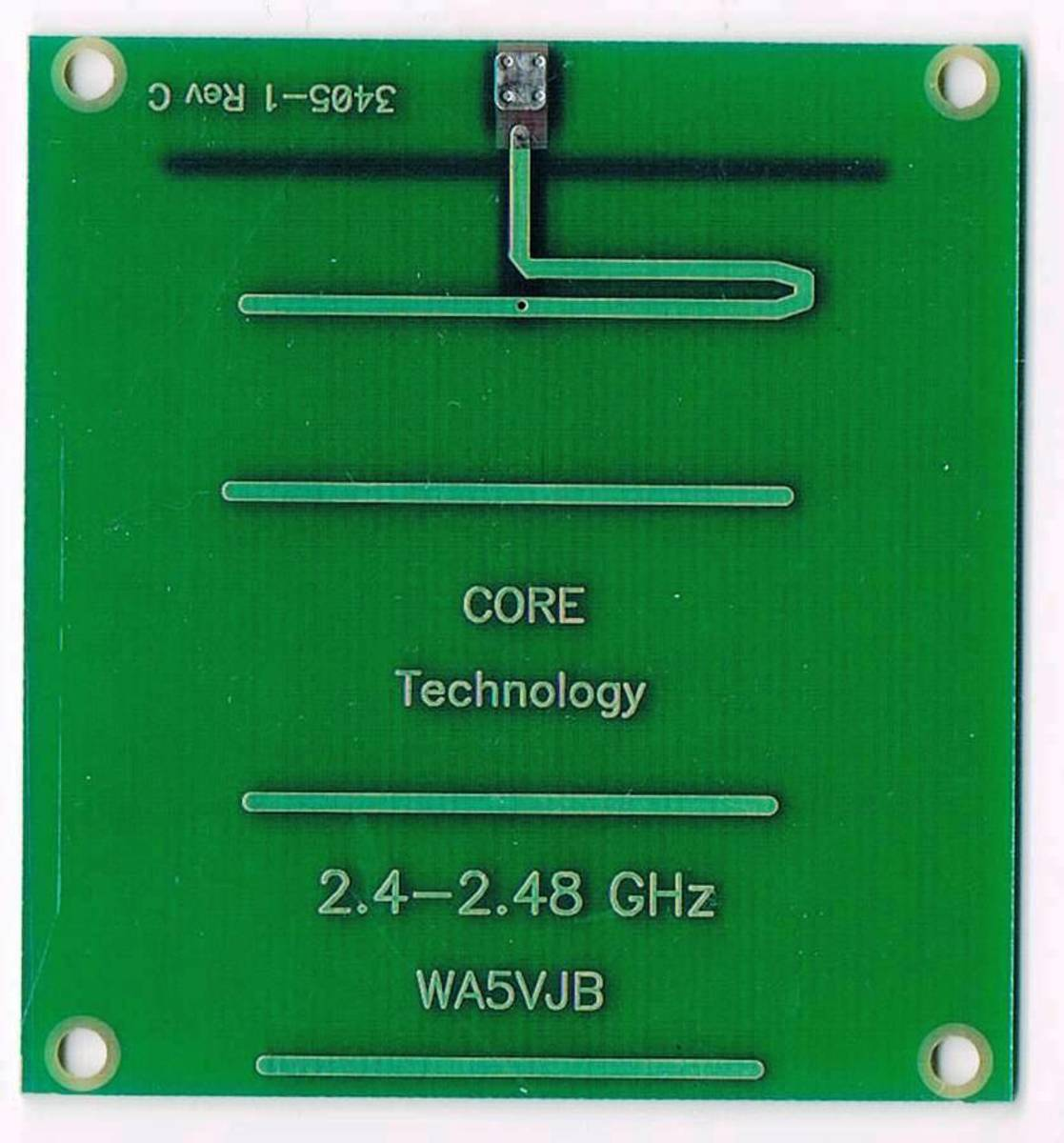# What Is the Quality Factor (Q Factor) of Antennas?

Tamara Wilhite is a technical writer, industrial engineer, mother of two, and published sci-fi and horror author.

## What Is the Q Factor of an Antenna?

The Q factor or quality factor of a tuned circuit (which includes antennas) measures how many times the current passes through the circuit. If an antenna has a Q factor of 10, then the current passes through its coils and capacitors ten times before the energy is radiated.

The Q factor of an antenna is a measure of the radiation efficiency of the antenna.

### Why Do We Use This Measure?

The attraction of using the Q factor as a measure of antenna performance is that it is dimensionless; dividing the center frequency used in the test by the difference between the 5:1 SWR points eliminates the units, yielding a clear ratio of 5, 10, 20 or higher for easy comparison between antennas, regardless of their frequency range.

## What Affects It?

Any resistance in the circuit hurts the Q factor. This means that in a high-Q antenna, a five ohm resistance from resistors begins to look like a 50-ohm dummy load (using the Q factor of 10).

With high-Q antennas, any loss not only lowers the Q but also widens the bandwidth of the antenna. To avoid this, loading coils for antennas and inductors are usually made from heavy gauge wire.

Low-Q antennas have low losses, and you often want to keep losses to a minimum. High-Q antennas are good if you are only receiving signals, since a high-Q antenna nearly filters out signals that are “out of band”. A low-Q antenna, on the other band, allows you to receive a wider bandwidth.

Antenna design affects the Q factor of an antenna. Patch antennas, as in the photo at the top of this article, are inherently high-Q antennas.

## How to Calculate the Q Factor of Your Antenna

The basic equation for the Q factor of an antenna is:

Q = (central frequency) ÷ (ƒ2 – ƒ1)

F2-F1 can be called the delta.

Scroll to Continue

### Finding F2 and F1

How do you find f2 and f1 to begin with?

1. Find an antenna tester or an amateur radio rig that can transmit a high SWR.
2. Remove the antenna tuner, if you have one, from the antenna you’re about to test. Set it at the antenna’s center frequency, such as 2.425 GHz for a 2.40 to 2.45 GHz antenna.
3. Tune down the tester until the SWR rises to 5:1. Note that point.
4. Then go up in frequency until the SWR rises to 5:1. Note the frequency where that ratio was reached.

You calculate Q by dividing the center frequency by the difference between the 5:1 bandwidth points.

### Example

Let’s say you have a center frequency of 2.4 GHz antenna. It reached the 5:1 SWR at 2.3 GHz and 2.5 GHz. The difference between the two bandwidth points is 2.5 GHz minus 2.3 GHz for 0.2 GHz. The center frequency of 2.4 GHz is divided by 0.2 GHz for an antenna Q of 12. In the case of the 2400 MHz antenna shown here with a 50 MHz frequency 1 and 2 delta, the Q factor is 48. There are log periodic antennas, on the other hand, with a Q factor of 1.

### Alternative Calculation Method

Another way to calculate Q is looking at the SWR curve for the antenna. You could use a 2:1 SWR as the definition for frequencies one and two.

1. Note the central frequency, the lowest point on the SWR curve. Then note the two points on the SWR curve where the SWR is 2.0.
2. Subtract those two frequencies from each other for the delta.
3. Divide the central frequency by that delta for the Q factor of the antenna.

These rough Q calculations don’t take the coax loss into account, but that makes the math much more difficult, so it will not be covered here. There are online calculators for determining the Q of an antenna with coax loss.This antenna has a narrow frequency range and Q factor approaching 50.Tamara Wilhite

## How to Change the Q Factor

A number of mobile antennas have loading coils made from heavy gauge wire in order to keep the resistive losses down, maximizing the Q factor. Cleaning the joints of the antenna can improve its efficiency, seen by a rise in the Q factor.

There is usually a tradeoff between Q factor and bandwidth—the broader the antenna’s bandwidth, the lower the Q factor. The higher the antenna loading, the higher Q factor and the narrower the bandwidth.

Shortening an antenna increases its Q factor. This is one of the benefits of using an antenna that is half a wavelength or quarter of a wavelength long over one that is the full wavelength of the intended signal frequency. For low frequencies, you have to use a ¼ wavelength antenna simply due to the size.